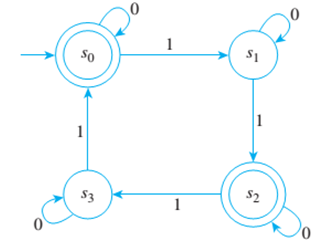Chapter 12.3, Problem 3ES### Discrete Mathematics With Applicat...

5th Edition
EPP + 1 other
ISBN: 9781337694193

#### Solutions

Chapter
Section### Discrete Mathematics With Applicat...

5th Edition
EPP + 1 other
ISBN: 9781337694193
Textbook Problem
1 views

# Consider the finite-state automaon A discussed in Example 12.3.1:a. Find the 0- and 1-equivalence classes of states of A.b. Draw the transition diagram of A ¯ , the quotient automaton of A.

To determine

(a)

Find the 0 and 1 equivalence classes of states of A.

Explanation

Given information:

The transition diagram of a finite state automaton A is given as follows.

Concept used:

Arrow in the given graph played vital role in answer selection.

Calculation:

It is required to rind the 0,1 and 2 equivalence classes of states of A.

0 Equivalence classes - Two states are zero equivalent if both are accepting states or both are non-accepting states. Therefore, the 0 equivalence classes are: {s1,s3},{s0,s2}

1 Equivalence classes — Two states are 1 equivalent if, and only if they are 0 equivalent and, after input of any symbol, their next state are 0 equivalent

To determine

(b)

Draw he transition diagram of A¯, the quotient automaton of A.

### Still sussing out bartleby?

Check out a sample textbook solution.

See a sample solution

#### The Solution to Your Study Problems

Bartleby provides explanations to thousands of textbook problems written by our experts, many with advanced degrees!

Get Started